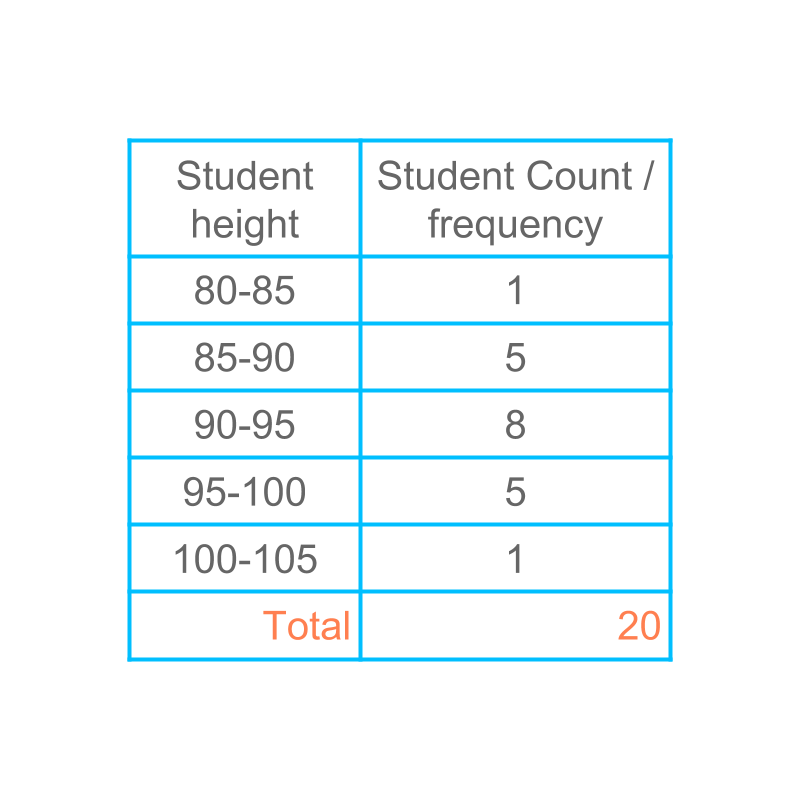maths > statistics-basics

Grouped Data - Class Interval & Class Mark

what you'll learn...

overview

In this page, class interval and class mark of grouped data is explained with some examples.

class intervalConsider the data given in the table. The height of $20$$20$ students are listed with number of students within the height range.

The number of students for each of the data value is called the frequency of that data value.

For example, the frequency of the class $85$$85$ to $90$$90$ is $5$$5$.

The range of the given data is $80$$80$ to $105$$105$.

In the given grouped data, the range $80$$80$ to $105$$105$ is divided into $5$$5$ classes.

The five classes are

•  student height from $80$$80$ to $85$$85$

•  student height from $85$$85$ to $90$$90$

•  student height from $90$$90$ to $95$$95$

•  student height from $95$$95$ to $100$$100$

•  student height from $100$$100$ to $105$$105$

Each of the classes is of the size $5$$5$. This size of division or the range of one class, is called the "class interval".

The word "interval" means: gap; space between two things.

class mark

In the given grouped data, the range $80$$80$ to $105$$105$ is divided into $5$$5$ classes.

The center point of each class interval is class mark of that class.

•  Class mark of $80-85$$80 - 85$ is $82.5$$82.5$

•  Class mark of $85-90$$85 - 90$ is $87.5$$87.5$

•  Class mark of $90-95$$90 - 95$ is $92.5$$92.5$

•  Class mark of $95-100$$95 - 100$ is $97.5$$97.5$

•  Class mark of $100-105$$100 - 105$ is $102.5$$102.5$

The word "mark" means: visible impression; indication of something.

Note that, the actual data values are not retained when the data is grouped into classes. The class mark is considered to be the representative value for all the grouped data in a class interval.

For example, the values of $81.2$$81.2$ and $84.6$$84.6$ are entered in the class $80$$80$ to $85$$85$. If the values are to be used for some computation (like computing mean), then instead of the actual values, the class mark $82.5$$82.5$ is used in their place.

summary

This size of division or the range of one class, is called the "class interval".

The center point of each class interval is class mark of that class.

Outline